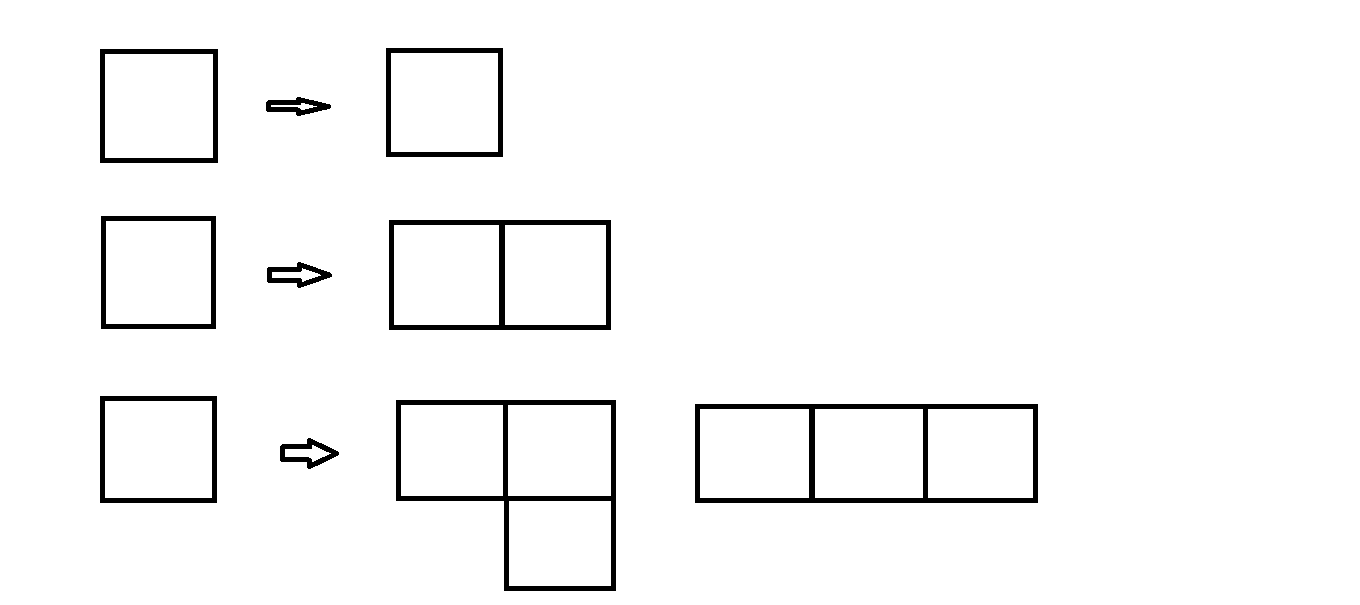# Counting SquaresLet us count the number of distinct shapes that can be formed using $n$ unit squares that are connected side to side. We consider rotations and reflections to be the same shape.

Consider the above image:
If we have 1 square, then it can only form 1 distinct shape.
If we have 2 squares, then it can only form 1 distinct shape.
If we have 3 squares, then it can form 2 distinct shapes.
If we have 4 squares, then they can form 5 distinct shapes. These are the straight line, the L-shape, the T-shape, the S-shape, and the box, which we see in a game of Tetris.

How many distinct shapes can be formed if we have 10 Squares ?

×